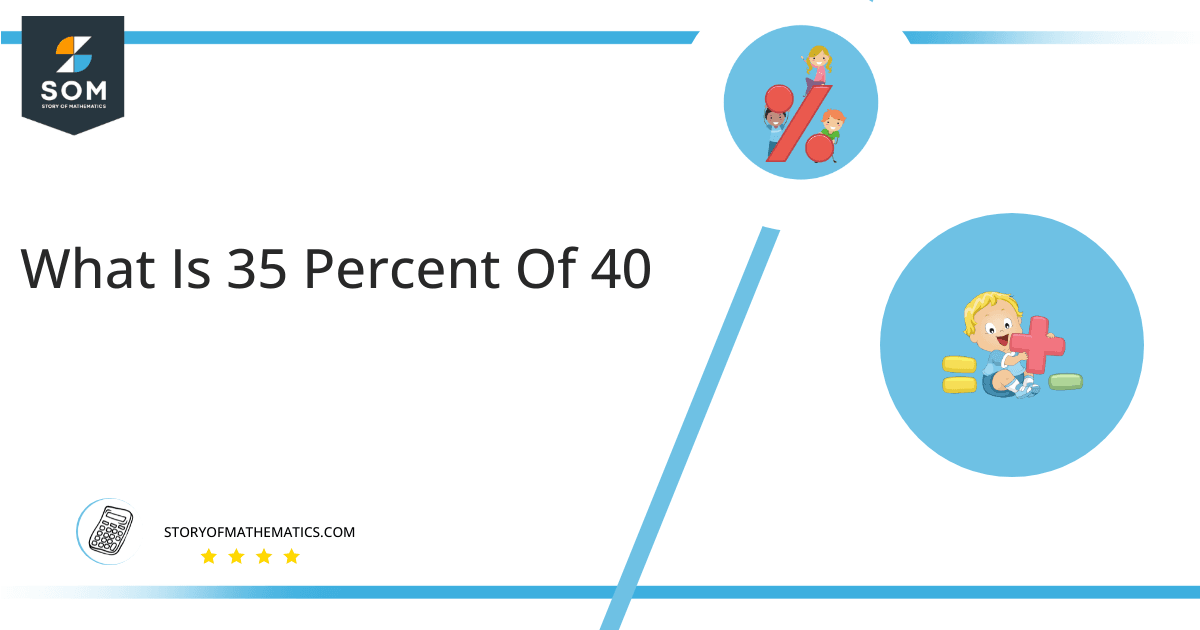# What Is 35 Percent of 40 + Solution with Free Steps?

The 35 percent of 40 is equal to 14. It can be easily calculated by dividing 35 by 100 and multiplying the answer with 40 to get 14.The easiest way to get this answer is by solving a simple mathematical problem of percentages. You need to find 35% of 40 for some sale or real-life problem. Divide 35 by 100, multiply the answer by 40, and get the 35% of 40 value in seconds.

This article will explain the full process of finding any percentage value from any given quantity or number with easy and simple steps.

## What Is 35 percent of 40?

The 35 percent of 40 is 14.

The percentage can be understood with a simple explanation. Take 40, and divide it into 100 equal parts. The 35 parts from the total of 100 parts is called 35 percent, which is 14 in this example.

## How To Calculate 35 percent of 40?

You can find 35 percent of 40 by some simple mathematical steps explained below.### Step 1

Firstly, depict 35 percent of 40 as a fractional multiple as shown below:

35% x 40

### Step 2

The percentage sign % means percent, equivalent to the fraction of 1/100.

Substituting this value in the above formula:

= (35/100) x 40

### Step 3

Using the algebraic simplification process, we can arithmetically manipulate the above equation as follows:

= (35 x 40) / 100

= 1400 / 100

= 14This percentage can be represented on a pie chart for visualization. Let us suppose that the whole pie chart represents the 40 values. Now, we find 35 percent of 40, which is 14. The area occupied by the 14 value will represent the 35 percent of the total 40 values. The remaining region of the pie chart will represent 65 percent of the total 40 values. The 100% of 40 will cover the whole pie chart as 40 is the total value.

Any given number or quantity can be represented in percentages to understand the total quantity better. The percentage can be considered a quantity that divides any number into hundred equal parts for better representation of large numbers and understanding.

Percentage scaling or normalization is a very simple and convenient method of representing numbers in relative terms. Such notations find wide application in many industrial sectors where the relative proportions are used.